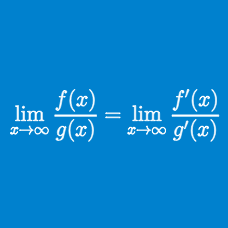Calculus

# L'Hopital's Rule: Level 2 Challenges

Please help me! I kept using L'Hôpital's rule millions of times and I can't evaluate the limit below!

$\displaystyle \lim_{x \to 0} \frac {\cot x }{\csc x } = \, ?$

Details and Assumptions:

• $\frac{d}{dx}( \cot x ) = -\csc^2 x$
• $\frac{d}{dx}( \csc x ) = -\csc x \cot x$

$\large \lim_{x \to 1}\frac {x^{n} + x^{n-1} + \ldots + x - n}{x - 1} = \ ?$

If the limit

$\lim _{ x\rightarrow 0 }{ \left( \frac { \sin { 2x } }{ { x }^{ 3 } } +a+\frac { b }{ { { x }^{ 2 } } } \right) } =0$

is true for constants $a$ and $b$, then what is the value of $3a+b?$

$\lim_{x \to 0} \left(\frac{1}{x^4} - \frac{ {\displaystyle\int_{0}^{x^2}} e^{-u^2} du}{x^6}\right) = \, ?$

What is the value of

$\lim _{ x \rightarrow 0 } \frac{ \tan x - x } { \sin x - x }?$

×

Problem Loading...

Note Loading...

Set Loading...